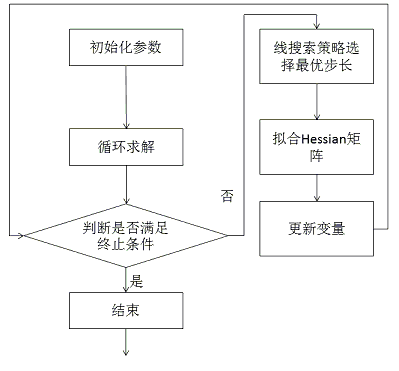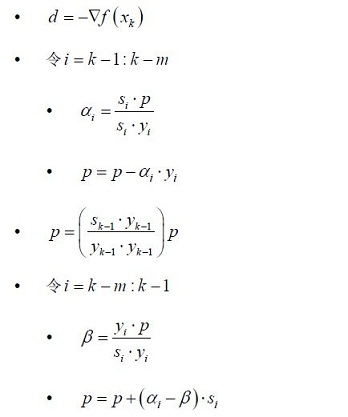# 机器学习算法实现解析——liblbfgs之L-BFGS算法

## 1、liblbfgs简介

liblbfgs是L-BFGS算法的C语言实现，用于求解非线性优化问题。

liblbfgs的主页：http://www.chokkan.org/software/liblbfgs/

## 2、liblbfgs源码解析

### 2.1、主要的结构

• liblbfgs-1.10/include/lbfgs.h：头文件
• liblbfgs-1.10/lib/lbfgs.c：具体的实现
• liblbfgs-1.10/lib/arithmetic_ansi.h(另两个arithmetic_sse_double.harithmetic_sse_float.h是两个汇编编写的等价形式)：相当于一些工具
• liblbfgs-1.10/sample/sample.c：测试样例

### 2.2、工具arithmetic_ansi.h

arithmetic_ansi.h文件中，主要是对向量（vector）的一些操作，这部分的程序代码比较简单，在这里简单对没个函数的作用进行描述，包括：

• 申请size大小的空间，同时对其进行初始化
void* vecalloc(size_t size)
• 释放空间
void vecfree(void *memblock)
• 将向量x中的值设置为c
void vecset(lbfgsfloatval_t *x, const lbfgsfloatval_t c, const int n)
• 将向量x中的值复制到向量y中
void veccpy(lbfgsfloatval_t *y, const lbfgsfloatval_t *x, const int n)
• 取向量x中的每个值的负数，将其放到向量y中
void vecncpy(lbfgsfloatval_t *y, const lbfgsfloatval_t *x, const int n)
• 对向量y中的每个元素增加向量x中对应元素的c倍
void vecadd(lbfgsfloatval_t *y, const lbfgsfloatval_t *x, const lbfgsfloatval_t c, const int n)
• 计算向量x和向量y的差
void vecdiff(lbfgsfloatval_t *z, const lbfgsfloatval_t *x, const lbfgsfloatval_t *y, const int n)
• 向量与常数的积
void vecscale(lbfgsfloatval_t *y, const lbfgsfloatval_t c, const int n)
• 向量的外积
void vecmul(lbfgsfloatval_t *y, const lbfgsfloatval_t *x, const int n)
• 向量的点积
void vecdot(lbfgsfloatval_t* s, const lbfgsfloatval_t *x, const lbfgsfloatval_t *y, const int n)
• 向量的点积的开方
void vec2norm(lbfgsfloatval_t* s, const lbfgsfloatval_t *x, const int n)
• 向量的点积的开方的倒数
void vec2norminv(lbfgsfloatval_t* s, const lbfgsfloatval_t *x, const int n)

### 2.3、L-BFGS算法的主要函数

#### 2.3.1、为变量分配和回收内存空间

// 为变量分配空间
lbfgsfloatval_t* lbfgs_malloc(int n)
{
// 涉及到汇编的一些知识，暂且不考虑
#if     defined(USE_SSE) && (defined(__SSE__) || defined(__SSE2__))
n = round_out_variables(n);
#endif/*defined(USE_SSE)*/
// 分配n个大小的内存空间
return (lbfgsfloatval_t*)vecalloc(sizeof(lbfgsfloatval_t) * n);
}

#if     LBFGS_FLOAT == 32
typedef float lbfgsfloatval_t;

#elif   LBFGS_FLOAT == 64
typedef double lbfgsfloatval_t;

#else
#error "libLBFGS supports single (float; LBFGS_FLOAT = 32) or double (double; LBFGS_FLOAT=64) precision only."

#endif

// 释放变量的空间
void lbfgs_free(lbfgsfloatval_t *x)
{
vecfree(x);
}

#### 2.3.2、L-BFGS中参数的初始化

// 默认初始化lbfgs的参数
void lbfgs_parameter_init(lbfgs_parameter_t *param)
{
memcpy(param, &_defparam, sizeof(*param));// 使用默认的参数初始化
}

#### 2.3.3、L-BFGS算法的核心过程概述

int n,// 变量的个数
lbfgsfloatval_t *x,// 变量
lbfgsfloatval_t *ptr_fx,// 目标函数值
lbfgs_evaluate_t proc_evaluate,// 计算目标函数值和梯度的回调函数
lbfgs_progress_t proc_progress,// 处理计算过程的回调函数
void *instance,// 数据
lbfgs_parameter_t *_param// L-BFGS的参数#### 2.3.5、循环求解的过程

/* Store the current position and gradient vectors. */
// 存储当前的变量值和梯度值
veccpy(xp, x, n);// 将当前的变量值复制到向量xp中
veccpy(gp, g, n);// 将当前的梯度值复制到向量gp中

/* Search for an optimal step. */
// 线搜索策略，搜索最优步长
if (param.orthantwise_c == 0.) {// 无L1正则
ls = linesearch(n, x, &fx, g, d, &step, xp, gp, w, &cd, &param);// gp是梯度
} else {// 包含L1正则
ls = linesearch(n, x, &fx, g, d, &step, xp, pg, w, &cd, &param);// pg是伪梯度
// 计算伪梯度
pg, x, g, n,
param.orthantwise_c, param.orthantwise_start, param.orthantwise_end
);
}
if (ls < 0) {// 已达到终止条件
// 由于在线搜索的过程中更新了向量x和向量g，因此从xp和gp中恢复变量值和梯度值
/* Revert to the previous point. */
veccpy(x, xp, n);
veccpy(g, gp, n);
ret = ls;
goto lbfgs_exit;// 释放资源
}

#### 2.3.6、循环的终止条件

• 是否收敛
vec2norm(&xnorm, x, n);// 平方和的开方
if (param.orthantwise_c == 0.) {// 非L1正则
vec2norm(&gnorm, g, n);
} else {// L1正则
vec2norm(&gnorm, pg, n);
}
// 判断是否收敛
if (xnorm < 1.0) xnorm = 1.0;
if (gnorm / xnorm <= param.epsilon) {
/* Convergence. */
ret = LBFGS_SUCCESS;
break;
}

|g(x)|max(1,|x|)<ε

• 目标函数值是否有足够大的下降（最小问题）
if (pf != NULL) {// 终止条件
/* We don't test the stopping criterion while k < past. */
// k为迭代次数，只考虑past>k的情况，past是指只保留past次的值
if (param.past <= k) {
/* Compute the relative improvement from the past. */
// 计算函数减小的比例
rate = (pf[k % param.past] - fx) / fx;

/* The stopping criterion. */
// 下降比例是否满足条件
if (rate < param.delta) {
ret = LBFGS_STOP;
break;
}
}

/* Store the current value of the objective function. */
// 更新新的目标函数值
pf[k % param.past] = fx;
}

pf中，保存了param.past次的目标函数值。计算的方法为：

fofnfn<Δ

• 是否达到最大的迭代次数
// 已达到最大的迭代次数
if (param.max_iterations != 0 && param.max_iterations < k+1) {
/* Maximum number of iterations. */
ret = LBFGSERR_MAXIMUMITERATION;
break;
}

#### 2.3.7、拟合Hessian矩阵

Hk+1=(IskyTkyTksk)THk(IyksTkyTksk)+sksTkyTkskL-BFGS的具体原理可以参见“优化算法——拟牛顿法之L-BFGS算法”。

• 计算向量序列 {sk} $\left \{ s_k \right \}$ {yk} $\left \{ y_k \right \}$
// 更新s向量和y向量
it = &lm[end];// 初始时，end为0
vecdiff(it->s, x, xp, n);// x - xp，xp为上一代的值，x为当前的值
vecdiff(it->y, g, gp, n);// g - gp，gp为上一代的值，g为当前的值
• 两个循环
// 通过拟牛顿法计算Hessian矩阵
// L-BFGS的两个循环
j = end;
for (i = 0;i < bound;++i) {
j = (j + m - 1) % m;    /* if (--j == -1) j = m-1; */
it = &lm[j];
/* \alpha_{j} = \rho_{j} s^{t}_{j} \cdot q_{k+1}. */
vecdot(&it->alpha, it->s, d, n);// 计算alpha
it->alpha /= it->ys;// 乘以rho
/* q_{i} = q_{i+1} - \alpha_{i} y_{i}. */
}

vecscale(d, ys / yy, n);

for (i = 0;i < bound;++i) {
it = &lm[j];
/* \beta_{j} = \rho_{j} y^t_{j} \cdot \gamma_{i}. */
vecdot(&beta, it->y, d, n);
beta /= it->ys;// 乘以rho
/* \gamma_{i+1} = \gamma_{i} + (\alpha_{j} - \beta_{j}) s_{j}. */
vecadd(d, it->s, it->alpha - beta, n);
j = (j + 1) % m;        /* if (++j == m) j = 0; */
}

vecdot(&ys, it->y, it->s, n);// 计算点积
vecdot(&yy, it->y, it->y, n);
it->ys = ys;

bound和end的计算方法如下所示：

bound = (m <= k) ? m : k;// 判断是否有足够的m代
++k;
end = (end + 1) % m;

## 补充

### 1、回调函数

• frame.h
#include <stdio.h>

void print_result(int (*get_result)(int, int), int a, int b){
printf("the final result is : %d\n", get_result(a, b));
}

• process.cc
#include <stdio.h>
#include "frame.h"

return a + b;
}

int sub(int a, int b){
return a - b;
}

int mul(int a, int b){
return a * b;
}

int max(int a, int b){
return (a>b?a:b);
}

int main(){
int a = 1;
int b = 2;
print_result(sub, a, b);
print_result(mul, a, b);
print_result(max, a, b);
return 1;
}

# 参考文献

05-2111-08139
11-24
09-132246
11-103万+
08-16
03-131万+
08-17258
04-221605
05-03650
04-271649
12-141105
01-052万+
07-275804
03-23490
01-152万+
03-241119
12-15
07-23